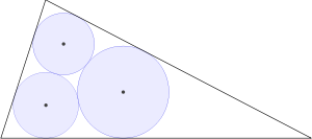# Revival of Sangaku 2

Geometry Level 5Let there be three mutually tangent circles of radius 6, 9, and 12. If a triangle inscribes the three circles (diagram not to scale), calculate the radius of the triangle's incircle.

×